# PowerPoint Matrix

### PowerPoint MatrixDescription:

PowerPoint matrix template set has a number of variants including 2×2 matrix and 3X3 matrix. The set has a number of 4 quadrant matrix charts including Market diversification matrixBCG matrix, Ansoff matrix, Product positioning matrix, decision matrix, information reporting matrix, comparison matrix, matrix with images, numbered matrix and 3D matrix. All the matrix diagrams are editable and professionally animated where required. The other keywords that indicate matrix diagrams in this set are: Boston matrix, Boston Consulting Group matrix, BCG analysis, consulting matrix, Ansoff model, Ansoff grid, decision matrix, criteria matrix etc.

Creative 2 X 2 Matrix in PowerPoint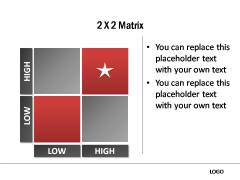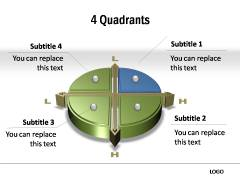Process Matrix | PowerPoint BCG Matrix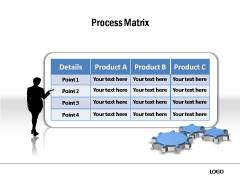Star / Question Mark/ Dog /Cash Cow in BCG Matrix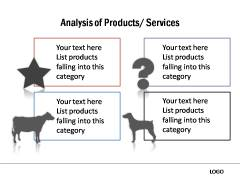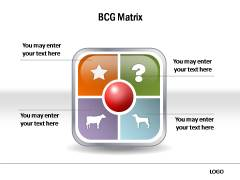Editable PowerPoint Table3D Table and Matrix to display data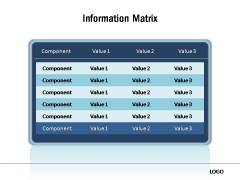Silver and Gold Framed PowerPoint Table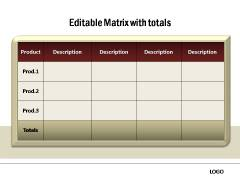Powerpoint Decision Matrix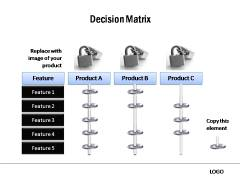Powerpoint Comparison MatrixPowerPoint Matrix Creative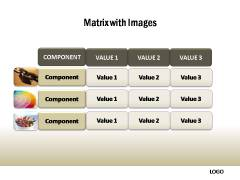RELATED DIAGRAMS IN THIS PACK

PowerPoint Calendar        |            PowerPoint Gantt